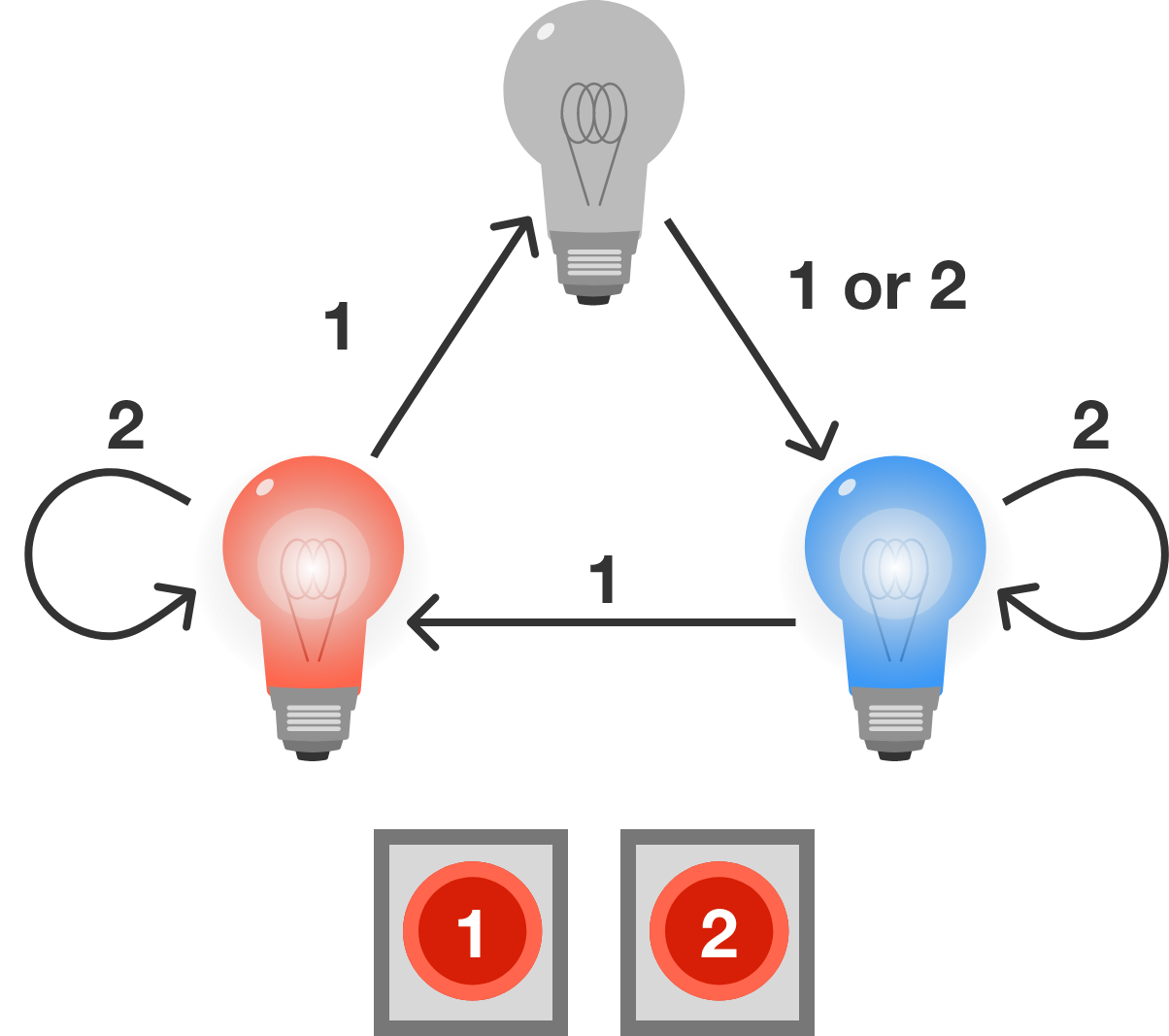# Turning off the light

Logic Level 1

There is a light in another room that can be in one of three states: $\color{#3D99F6}\text{blue},$ $\color{#D61F06}\text{red},$ or $\text{off}.$

In your room, you have two buttons numbered 1 and 2 that control the state of the light. The state of light changes according to the diagram below.• If you press button 1, then the light changes to the next state clockwise around the diagram.
• If you press button 2 while the light is $\color{#3D99F6}\text{blue}$ or $\color{#D61F06}\text{red},$ the state of the light will not change.
• If you press button 2 while the light is $\text{off},$ the light will change to $\color{#3D99F6}\text{blue}.$

You want to make sure that the light is $\text{off},$ but since the light is in another room, you do not know what state the light is currently in.

What is the shortest sequence of button presses that will guarantee that the light will be $\text{off}?$

Note: Enter your answer as a sequence of digits in order. For example, if you believe the sequence is {1, 2, 1, 2, 1}, then enter $12121.$

×